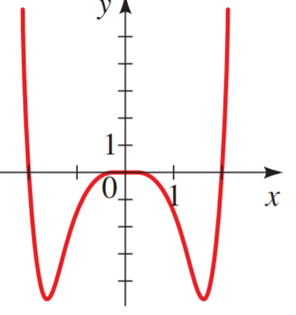# To match : the polynomial function### Precalculus: Mathematics for Calcu...

6th Edition
Stewart + 5 others
Publisher: Cengage Learning
ISBN: 9780840068071### Precalculus: Mathematics for Calcu...

6th Edition
Stewart + 5 others
Publisher: Cengage Learning
ISBN: 9780840068071

#### Solutions

Chapter 3.2, Problem 12E
To determine

## To match: the polynomial function

Expert Solution### Explanation of Solution

Given information:

The polynomial function is S(x)=12x62x4

The power of the equation is even and the leading co-efficient positiveso, predict the end behaviour, which is when x approaches positive , y will approach positive and as x approaches negative , y will approach positive .so, graph IIand VI contain this characteristic. Now substitute x with any value

12162(1)4                          [substitute the value of x]122                                        [evaluate power]0.52                                     [divide]1.5                                           [subtract]

Therefore ,the coordinate point (1, 1.5 ),the graph II contain this closest

Hence, the correct answer is II.

### Have a homework question?

Subscribe to bartleby learn! Ask subject matter experts 30 homework questions each month. Plus, you’ll have access to millions of step-by-step textbook answers!